# For the reversible reaction which k values would indicate that there is more B than A at equilibrium?

For the reversible reaction which k values would indicate that there is more B than A at equilibrium?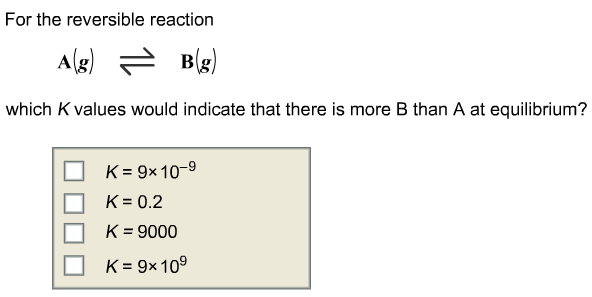Concepts and reason
The concept used to solve this problem is based on the equilibrium constant of a chemical reaction.

The value of the reaction quotient when a reaction reaches equilibrium is called equilibrium constant. It is the ratio of the concentrations of products and concentrations of reactants.

Fundamentals
For a chemical reaction,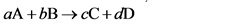The equilibrium constant is written as follow.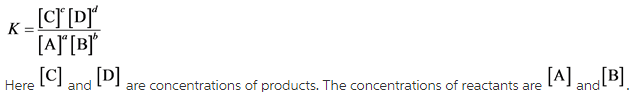For the reaction,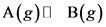The equilibrium constant is written as follow.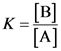Explanation:
The equilibrium constant is the ratio of the concentrations of products to the concentration of reactants.

The values of K, 0.2 and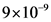are less than one. Thus, at these values the reactant A is more than product B at equilibrium.

Explanation:
If the value of K is less than one then there is more reactant than product at equilibrium and if the value of K is more than one than there is more product than reactant at equilibrium.

The values of K, 9000 andare more than one. Thus, at these values the product B is more than reactant A at equilibrium.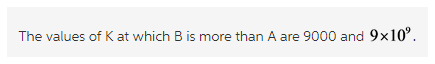Explanation:
The equilibrium constant is written as the ratio of concentrations of products and concentrations of reactants. The product formed is more when the value of equilibrium constant is more than one.